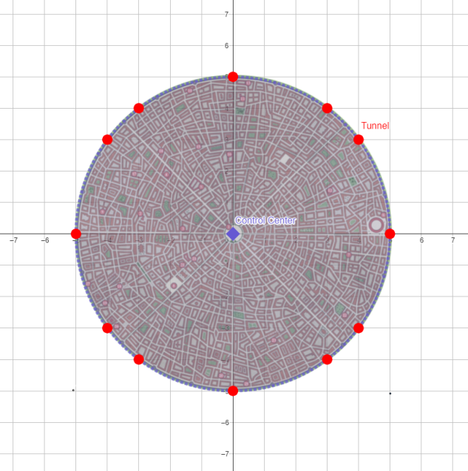# AI Empiren3wb13_223DIU Take-Off Programming...1/7/28
Limits 4s, 512 MB

This is the year $3202$. $300$ years ago, AI got so much smart that AI took control over its creators. Now A war is running between Humans and AI, where AI is more powerful than humans. Because all of the advanced technologies belong to AI. Humans have to steal those technologies to fight against AI. But it's quite impossible to enter the AI empire because of its tight security system. If someone gets caught, he/she won't be alive. So this is also a huge risk to enter in AI empire to steal its technologies.

Fortunately, we have a complete map of the AI empire. After analyzing this map several times, we got some key information about the AI arena. If we consider the AI empire in a Cartesian coordinate system, then

1. The empire is a circular area with radius $R$.

2. The control center is located at point $(0,0)$, exactly in the center of AI empire.

3. There is a radar located at the control center that covers the full area with a radius $R$.

Currently, We are running out of guns and power sources. That's why we have to manage some stuff from the AI empire. After analyzing this map, we suddenly discovered a piece of new key information.

Several tunnels exist at the AI empire's border, located at lattice points only. This tunnel goes straight to the AI control center. So we want as many soldiers as possible to enter the AI control center. For this, first, we have to know how many tunnels exist at the border.

The point having integer coordinates is called a lattice point.

Below is a map of an AI Empire having $5$ units radius.The control center is at $(0,0)$.

There are $12$ tunnels whose coordinates are,

1. $(5,0)$

2. $(4,3)$

3. $(3,4)$

4. $(0,5)$

5. $(-3,4)$

6. $(-4,3)$

7. $(-5,0)$

8. $(-4,-3)$

9. $(-3,-4)$

10. $(0,-5)$

11. $(3,-4)$

12. $(4,-3)$

It can be seen that all of the tunnels are situated on the empire’s border and also lattice points.

As AI is against us, we need someone who can tell us the total number of tunnels at the border with the information mentioned above. Being on our side, can you solve this problem for us?

## Input

The first line contains a single integer $t$ $(1 \le t \le 10^6)$ — the number of test cases.

The only line of each test case contains an integer $R$ $(1 \le R \le 10^6)$ — the radius of the AI empire.

## Output

For each test case, output a single integer — the number of tunnels that exist at the AI empire’s border.

## Sample

InputOutput
4
2
3
4
5

4
4
4
12


### Submit